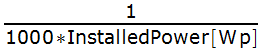# Relative powers

## Contents

If you have for example more than one solar plant/inverter with different size/orientation, the absolute power values in watt are not so helpful for comparison.

A common unit for comparison is kilo watt per kilo watt peak.

So therefor you have to transform the watt data from a power sensor into kilo watt per kilo watt peak.

## Setup

• A power sensor (with unit watt in this example)
• A proxy channel for calculation

I use for this the calculator channel type, which is meant for such a use case.

To force the proxy to make the calculation, a proper resolution must be set.

• Inverter 1 installed power: 11.28 kilo watt peak
• Inverter 2 installed power: 9.18 kilo watt peak

Transform watt into kilo watt per kilo watt peak:## Channel definitions

Values for the factor attributes:

• Inverter 1: 0.0000887
• Inverter 2: 0.000109

Build a proper channel hierarchy:

## Chart

Now the channels are better comparable: